# Teaching Perpendicular and Angle Bisectors

In geometry, students learn about perpendicular and angle bisectors. They become proficient in applying theorems about perpendicular bisectors, as well as in applying theorems about angle bisectors.

To make perpendicular and angle bisector lessons more interesting, math teachers and homeschooling parents can employ diverse teaching strategies, including more engaging teaching and interactive games. Read on to learn more.

## Perpendicular and Angle Bisector Teaching Strategies

### What Is a Bisector?

Explain to students that a bisector represents the thing that cuts (or bisects) an object into two equal parts. For instance, a bisector can bisect angles, line segments, etc. To present an example, you can simply draw a line segment and then a line that bisects it into two equal parts.

### What Are Equidistant Points?

Students are already familiar with what a point means. Explain that we say that a point is equidistant from a set of objects if the distances between that point and each object in the mentioned set are equal.### What Is the Perpendicular Bisector Theorem?

Before you explain the perpendicular bisector theorem, point out that perpendicularity refers to the relationship between two lines that meet at angles of 90 degrees. So perpendicular lines are lines that form right angles when they intersect.

Present an example of two lines that are perpendicular, such as: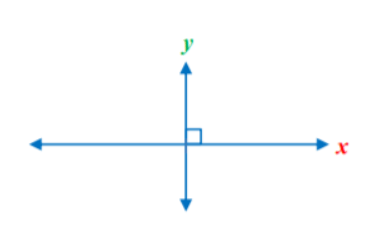Add that line x is perpendicular to line y because their intersection results in right angles. Now you can proceed with explaining what a perpendicular bisector means. This is a segment, a ray, line, or a plane that is perpendicular to a segment at its midpoint.

Then, remind students that the midpoint of a line segment is the point that divides the segments into two equal parts, or simply the middle point of a segment. Draw an example of a perpendicular bisector on the whiteboard: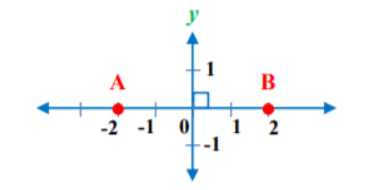Add that in this drawing, line y is the perpendicular bisector of the line segment AB, because this line intersects the segments at right angles.

Now that students understand perpendicular bisectors and equidistant points, you can explain the perpendicular bisector theorem. This theorem states that if a point is on the perpendicular bisector of a segment, then it is equidistant from the endpoints of a segment.

Present a drawing to illustrate the perpendicular bisector theorem, such as the drawing below: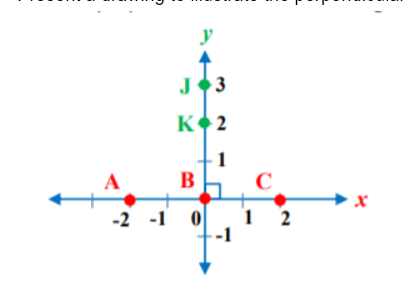Point out we can see that the points J and K are on the perpendicular bisector of the line segment AC. According to the theorem, the points J and K are equidistant from the endpoints of the line segment AC.

### The Converse of the Perpendicular Bisector Theorem

Explain that the converse of the perpendicular bisector theorem also holds true. It states that if a point is equidistant from the endpoints of a segment, then it is on the perpendicular bisector of a segment. So in terms of the above drawing, we can also say that: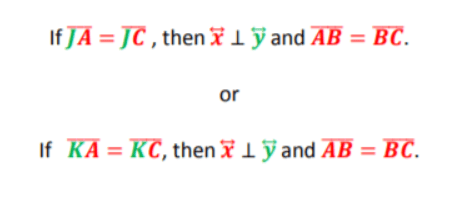### What Is the Angle Bisector Theorem?

Explain to students that in addition to the perpendicular bisector theorem, there’s also an angle bisector theorem. Before explaining this theorem, you can first explain that an angle bisector represents a segment, a ray, line, or a plane dividing the angle into two equal parts.

Draw an angle bisector, such as the one below, and add that the angle bisector of the angle BAD is the ray AC: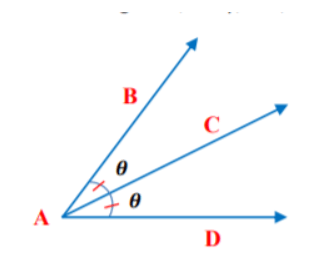Then, point out that the angle bisector theorem states that if a point is on the bisector of an angle, then it is equidistant from the sides of the angle. In the drawing below, point C is on the angle bisector, which means that the length of line BC equals line CD.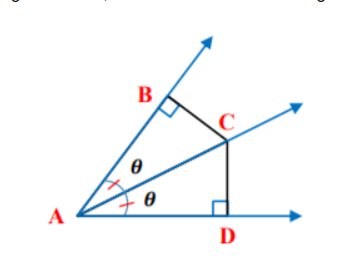### The Converse of the Angle Bisector Theorem

Explain to students that if we reverse the statements of the angle bisector theorem, it will result in the converse of the angle bisector theorem. According to this theorem, if a point in the interior of an angle is equidistant from the sides of the angle, then it’s on the bisector of the angle.

Thus, in terms of the above drawing, we can also say that:## Perpendicular and Angle Bisector Activities

### Triangles and Bisectors Game

This is an online game that will help students practice recognizing bisectors, and using the angle bisector theorem. To use this game in your classroom, make sure there is a suitable technical device for each student.

Students play the game individually. They are presented with a number of questions related to bisectors. Provide a few minutes for this and then open a discussion. Were any of the questions more challenging? How did the angle bisector theorem help them in answering the questions?

### Group Work

This is a group activity that will help students strengthen their knowledge of the perpendicular bisector theorem, as well as the angle bisector theorem. To implement this activity in your classroom, you’ll need to print out this Assignment Worksheet (Members Only).

Divide students into groups of 3 or 4 and hand out the worksheets. Explain to students that the worksheets contain several questions related to the two theorems. Students work together with the other members of their group to answer the questions.

In the end, each group presents at least one exercise in front of the class. They explain how they solved it, and how the perpendicular bisector theorem and the angle bisector theorem helped them in the process.

## Before You Leave…

If you liked our strategies on teaching perpendicular and angle bisectors, and you’re looking for more math resources for kids of all ages, sign up for our emails to receive loads of free resources, including worksheets, guided lesson plans and notes, activities, and much more!

Feel free to also head over to our blog, where you’ll find plenty of free and awesome resources! And if you’d like to become a member, join us in the Math Teacher Coach community!